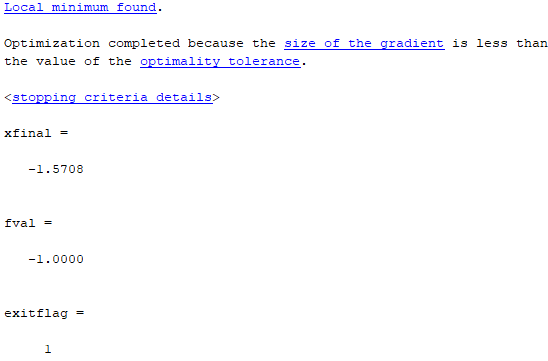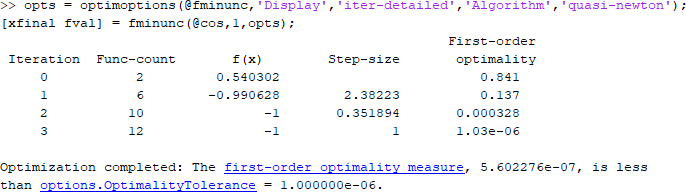Documentation

## Exit Flags and Exit Messages

### Exit Flags

When an optimization solver completes its task, it sets an exit flag. An exit flag is an integer that is a code for the reason the solver halted its iterations. In general:

• Positive exit flags correspond to successful outcomes.

• Negative exit flags correspond to unsuccessful outcomes.

• The zero exit flag corresponds to the solver being halted by exceeding an iteration limit or limit on the number of function evaluations (see Iterations and Function Counts, and also see Tolerances and Stopping Criteria).

A table of solver outputs in the solver's function reference section lists the meaning of each solver's exit flags.

### Note

Exit flags are not infallible guides to the quality of a solution. Many other factors, such as tolerance settings, can affect whether a solution is satisfactory to you. You are responsible for deciding whether a solver returns a satisfactory answer. Sometimes a negative exit flag does not correspond to a “bad” solution. Similarly, sometimes a positive exit flag does not correspond to a “good” solution.

You obtain an exit flag by calling a solver with the `exitflag` syntax. This syntax depends on the solver. For details, see the solver function reference pages. For example, for `fsolve`, the calling syntax to obtain an exit flag is

`[x,fval,exitflag] = fsolve(...)`

The following example uses this syntax. Suppose you want to solve the system of nonlinear equations

`$\begin{array}{c}2{x}_{1}-{x}_{2}={e}^{-{x}_{1}}\\ -{x}_{1}+2{x}_{2}={e}^{-{x}_{2}}.\end{array}$`

Write these equations as an anonymous function that gives a zero vector at a solution:

```myfcn = @(x)[2*x(1) - x(2) - exp(-x(1)); -x(1) + 2*x(2) - exp(-x(2))];```

Call `fsolve` with the `exitflag` syntax at the initial point [-5 -5]:

```[xfinal fval exitflag] = fsolve(myfcn,[-5 -5]) Equation solved. fsolve completed because the vector of function values is near zero as measured by the value of the function tolerance, and the problem appears regular as measured by the gradient. xfinal = 0.5671 0.5671 fval = 1.0e-06 * -0.4059 -0.4059 exitflag = 1```

In the table for `fsolve` `exitflag`, you find that an exit flag value `1` means “Function converged to a solution `x`.” In other words, `fsolve` reports `myfcn` is nearly zero at `x` = `[0.5671 0.5671]`.

### Exit Messages

Each solver issues a message to the MATLAB® command window at the end of its iterations. This message explains briefly why the solver halted. The message might give more detail than the exit flag.

Many examples in this documentation show exit messages. For example, see Minimize Rosenbrock's Function at the Command Line, or Step 3: Invoke fminunc using the options.. The example in the previous section, Exit Flags, shows the following exit message:

```Equation solved. fsolve completed because the vector of function values is near zero as measured by the value of the function tolerance, and the problem appears regular as measured by the gradient.```

This message is more informative than the exit flag. The message indicates that the gradient is relevant. The message also states that the function tolerance controls how near 0 the vector of function values must be for `fsolve` to regard the solution as completed.

### Enhanced Exit Messages

• Links on words or phrases. If you click such a link, a window opens that displays a definition of the term, or gives other information. The new window can contain links to the Help browser documentation for more detailed information.

• A link as the last line of the display saying ```<stopping criteria details>```. If you click this link, MATLAB displays more detail about the reason the solver halted.

The `fminunc` solver has enhanced exit messages:

```opts = optimoptions(@fminunc,'Algorithm','quasi-newton'); % 'trust-region' needs gradient [xfinal fval exitflag] = fminunc(@sin,0,opts)```

This yields the following results:• The `<stopping criteria details>` link prints the following to the MATLAB command line:

```Optimization completed: The first-order optimality measure, 0.000000e+00, is less than options.OptimalityTolerance = 1.000000e-06.```
• The other links bring up a help window with term definitions. For example, clicking the `Local minimum found` link opens the following window:Clicking the `first-order optimality measure` expander link brings up the definition of first-order optimality measure for `fminunc`:The expander link is a way to obtain more information in the same window. Clicking the `first-order optimality measure` expander link again closes the definition.

• The other links open the Help Viewer.

### Exit Message Options

Set the `Display` option to control the appearance of both exit messages and iterative display. For more information, see Iterative Display. The following table shows the effect of the various settings of the `Display` option.

Value of the Display OptionOutput to Command Window
Exit messageIterative Display
`'none'`, or the synonymous `'off'`NoneNone
`'final'` (default for most solvers)DefaultNone
`'final-detailed'`DetailedNone
`'iter'`DefaultYes
`'iter-detailed'`DetailedYes
`'notify'`Default only if `exitflag` ≤ 0None
`'notify-detailed'`Detailed only if `exitflag` ≤ 0None

For example,

```opts = optimoptions(@fminunc,'Display','iter-detailed','Algorithm','quasi-newton'); [xfinal fval] = fminunc(@cos,1,opts);```

yields the following display: